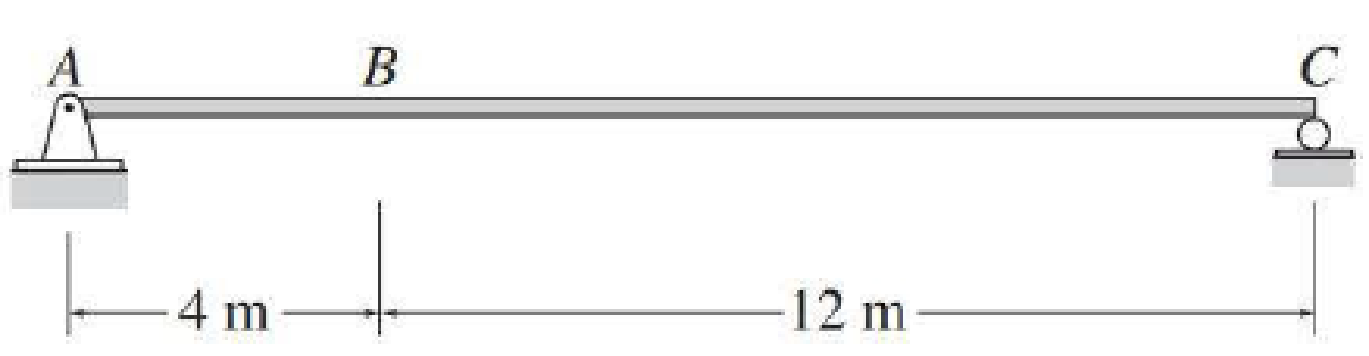# 8.59 and 8.60 Draw the influence line for the vertical deflection at point B of the simply supported beams of Problems 8.1 and 8.2. EI = constant. See Figs. P8.1 and P8.2. FIG. P8.2, P8.60

#### Solutions

Chapter
Section
Chapter 8, Problem 60P
Textbook Problem
3 views

## 8.59 and 8.60 Draw the influence line for the vertical deflection at point B of the simply supported beams of Problems 8.1 and 8.2. EI = constant. See Figs. P8.1 and P8.2.FIG. P8.2, P8.60

To determine

Sketch the influence line for the vertical deflection at the point B of the simply supported beam.

### Explanation of Solution

Given information:

Calculation:

Consider a point load is acting at a distance x from the left support of the beam.

Show the loading on the simply supported beam as shown in Figure 1.

Refer Figure 1.

Consider the beam portion ranges from 0mx4m.

Show the expression for the deflection of the simply supported beam due to point load P acting at a distance a from the left support as follows:

Δ=Pbx6lEI(l2x2b2),0<x<a        (1)

Here, a and b are the location of the point load from the left and right support, and l is the length of the beam.

Calculate the deflection (fBX) of the simply supported beam at point B due to the unit point load acting at a distance x from the left support.

Substitute 1 for P, 16m for l, and 12m for b in Equation (1).

fBX=1×12×x6×16EI(162x2122)fBX=18EI(112xx3)        (2)

Consider the load is acting at a distance x as 2m.

Calculate the deflection at B as follows:

Substitute 2m for x in Equation (2).

fBX=18EI(112×223)fBX=27EI

Similarly, calculate the deflection at point B due to different position of the point load and tabulate the values as shown in Table 1.

 x (m) fBX 2 −27EI 3 −38.625EI 4 −48EI

Consider the beam portion ranges from 4mx16m.

Show the expression for the deflection of the simply supported beam due to point load P acting at a distance a from the left support as follows:

Δ=Pb6lEI[lb(xa)3+(l2b2)xx3],a<x<l        (3)

Here, l is the distance between the fixed end and the section where the deflection is required, E is modulus of elasticity of the beam, and I is the moment of inertia.

Calculate the deflection (fBX) of the simply supported beam at point B due to the unit point load acting at a distance x from the left supported

### Still sussing out bartleby?

Check out a sample textbook solution.

See a sample solution

#### The Solution to Your Study Problems

Bartleby provides explanations to thousands of textbook problems written by our experts, many with advanced degrees!

Get Started

Find more solutions based on key concepts
VocabularyState the meaning of the following terms: Steel _____ Bronze _____ Brass _____ 18/8 Stainless Steel _...

Engineering Fundamentals: An Introduction to Engineering (MindTap Course List)

In Oracle, what is a database profile?

Database Systems: Design, Implementation, & Management

What is a DataSet, and why is it considered to be disconnected?

Database Systems: Design, Implementation, & Management

Name and give examples of the components of a web address.

Enhanced Discovering Computers 2017 (Shelly Cashman Series) (MindTap Course List)

What is XML, and how is it used?

Fundamentals of Information Systems

What other term is used to refer to the OFC process?

Welding: Principles and Applications (MindTap Course List)

If your motherboard supports ECC DDR3 memory, can you substitute non-ECC DDR3 memory?

A+ Guide to Hardware (Standalone Book) (MindTap Course List)